# Selina Solutions Concise Mathematics Class 6 Chapter 22 Simple (Linear) Equations Exercise 22(A)

Selina Solutions Concise Mathematics Class 6 Chapter 22 Simple (Linear) Equations Exercise 22(A) provides students comprehensive answers to each and every question, with illustrations. The solutions are formulated by faculty after conducting research on each concept, in accordance with the understanding capacity of students. Referring to these solutions, students can clear their doubts quickly, which arise while solving exercise problems. Here, students can download Selina Solutions Concise Mathematics Class 6 Chapter 22 Simple (Linear) Equations Exercise 22(A) free PDF, from the links which are given below.

## Selina Solutions Concise Mathematics Class 6 Chapter 22 Simple (Linear) Equations Exercise 22(A) Download PDF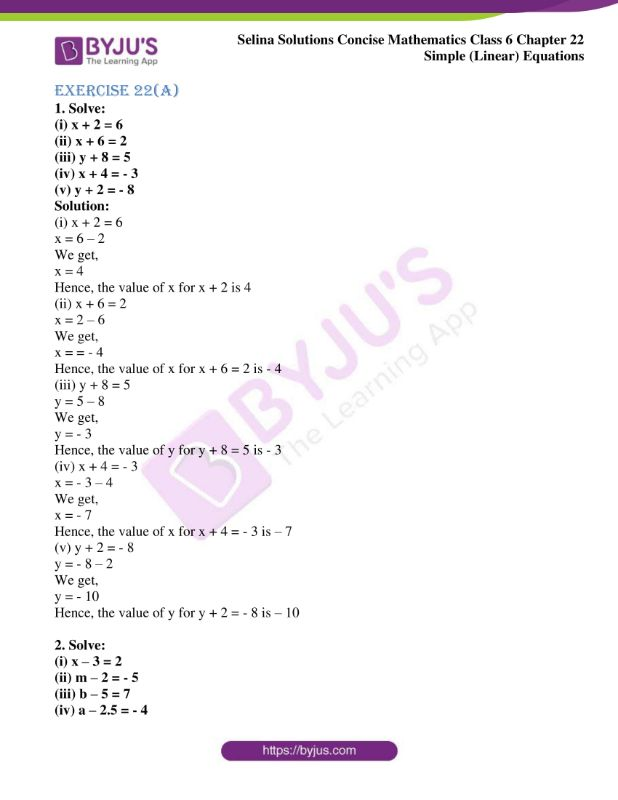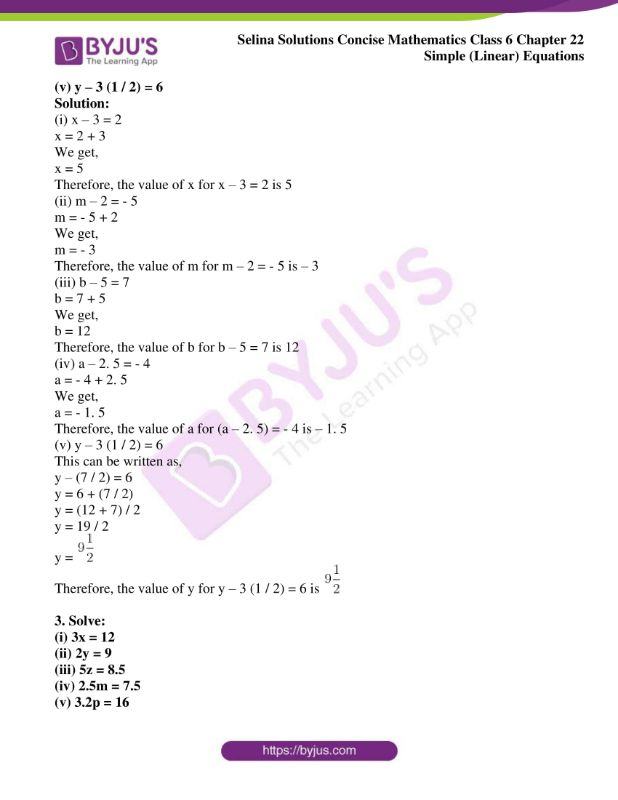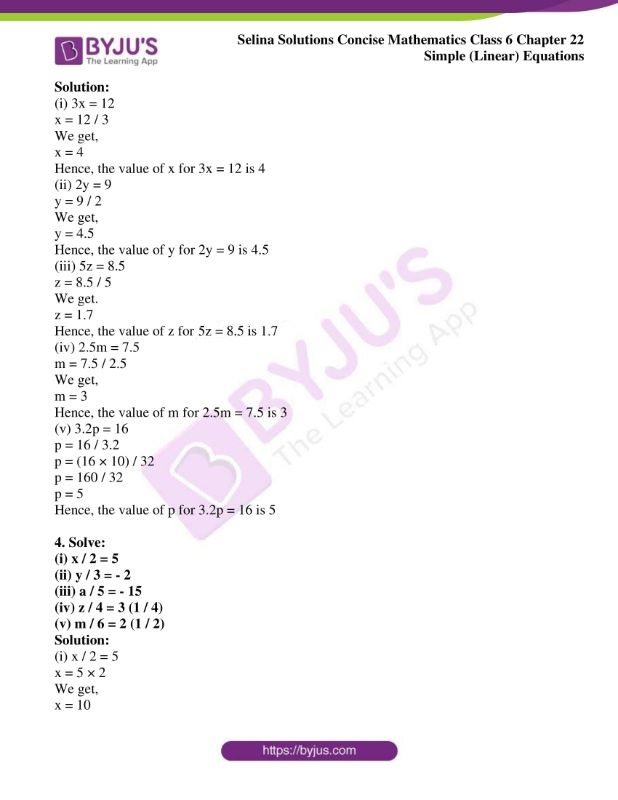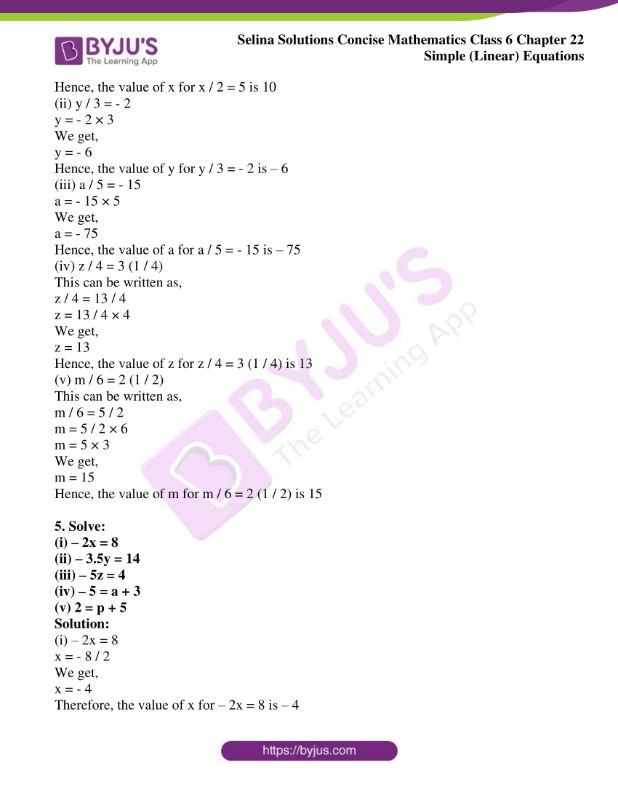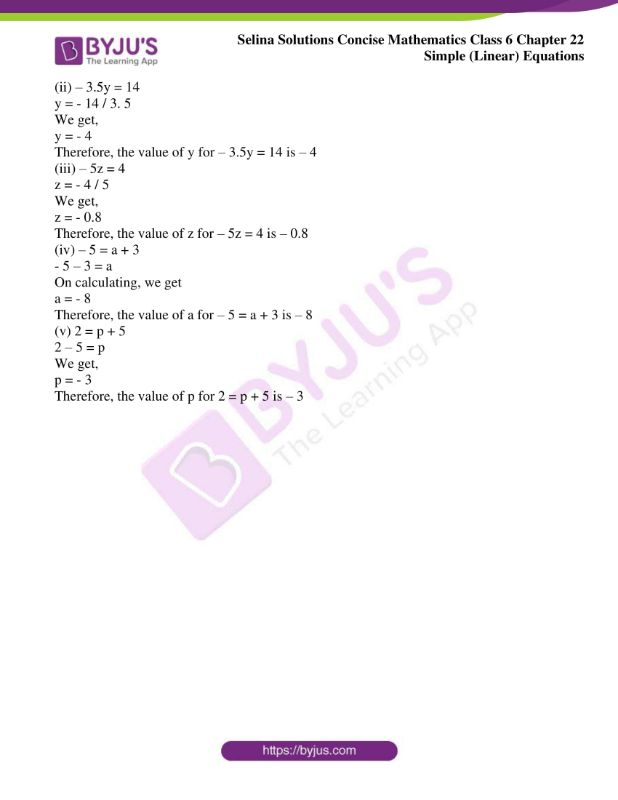### Access other exercises of Selina Solutions Concise Mathematics Class 6 Chapter 22 Simple (Linear) Equations

Exercise 22(B) Solutions

Exercise 22(C) Solutions

Exercise 22(D) Solutions

### Access Selina Solutions Concise Mathematics Class 6 Chapter 22: Simple (Linear) Equations Exercise 22(A)

Exercise 22(A)

1. Solve:

(i) x + 2 = 6

(ii) x + 6 = 2

(iii) y + 8 = 5

(iv) x + 4 = – 3

(v) y + 2 = – 8

Solution:

(i) x + 2 = 6

x = 6 – 2

We get,

x = 4

Hence, the value of x for x + 2 is 4

(ii) x + 6 = 2

x = 2 – 6

We get,

x = = – 4

Hence, the value of x for x + 6 = 2 is – 4

(iii) y + 8 = 5

y = 5 – 8

We get,

y = – 3

Hence, the value of y for y + 8 = 5 is – 3

(iv) x + 4 = – 3

x = – 3 – 4

We get,

x = – 7

Hence, the value of x for x + 4 = – 3 is – 7

(v) y + 2 = – 8

y = – 8 – 2

We get,

y = – 10

Hence, the value of y for y + 2 = – 8 is – 10

2. Solve:

(i) x – 3 = 2

(ii) m – 2 = – 5

(iii) b – 5 = 7

(iv) a – 2.5 = – 4

(v) y – 3 (1 / 2) = 6

Solution:

(i) x – 3 = 2

x = 2 + 3

We get,

x = 5

Therefore, the value of x for x – 3 = 2 is 5

(ii) m – 2 = – 5

m = – 5 + 2

We get,

m = – 3

Therefore, the value of m for m – 2 = – 5 is – 3

(iii) b – 5 = 7

b = 7 + 5

We get,

b = 12

Therefore, the value of b for b – 5 = 7 is 12

(iv) a – 2. 5 = – 4

a = – 4 + 2. 5

We get,

a = – 1. 5

Therefore, the value of a for (a – 2. 5) = – 4 is – 1. 5

(v) y – 3 (1 / 2) = 6

This can be written as,

y – (7 / 2) = 6

y = 6 + (7 / 2)

y = (12 + 7) / 2

y = 19 / 2

y =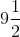Therefore, the value of y for y – 3 (1 / 2) = 6 is3. Solve:

(i) 3x = 12

(ii) 2y = 9

(iii) 5z = 8.5

(iv) 2.5m = 7.5

(v) 3.2p = 16

Solution:

(i) 3x = 12

x = 12 / 3

We get,

x = 4

Hence, the value of x for 3x = 12 is 4

(ii) 2y = 9

y = 9 / 2

We get,

y = 4.5

Hence, the value of y for 2y = 9 is 4.5

(iii) 5z = 8.5

z = 8.5 / 5

We get.

z = 1.7

Hence, the value of z for 5z = 8.5 is 1.7

(iv) 2.5m = 7.5

m = 7.5 / 2.5

We get,

m = 3

Hence, the value of m for 2.5m = 7.5 is 3

(v) 3.2p = 16

p = 16 / 3.2

p = (16 × 10) / 32

p = 160 / 32

p = 5

Hence, the value of p for 3.2p = 16 is 5

4. Solve:

(i) x / 2 = 5

(ii) y / 3 = – 2

(iii) a / 5 = – 15

(iv) z / 4 = 3 (1 / 4)

(v) m / 6 = 2 (1 / 2)

Solution:

(i) x / 2 = 5

x = 5 × 2

We get,

x = 10

Hence, the value of x for x / 2 = 5 is 10

(ii) y / 3 = – 2

y = – 2 × 3

We get,

y = – 6

Hence, the value of y for y / 3 = – 2 is – 6

(iii) a / 5 = – 15

a = – 15 × 5

We get,

a = – 75

Hence, the value of a for a / 5 = – 15 is – 75

(iv) z / 4 = 3 (1 / 4)

This can be written as,

z / 4 = 13 / 4

z = 13 / 4 × 4

We get,

z = 13

Hence, the value of z for z / 4 = 3 (1 / 4) is 13

(v) m / 6 = 2 (1 / 2)

This can be written as,

m / 6 = 5 / 2

m = 5 / 2 × 6

m = 5 × 3

We get,

m = 15

Hence, the value of m for m / 6 = 2 (1 / 2) is 15

5. Solve:

(i) – 2x = 8

(ii) – 3.5y = 14

(iii) – 5z = 4

(iv) – 5 = a + 3

(v) 2 = p + 5

Solution:

(i) – 2x = 8

x = – 8 / 2

We get,

x = – 4

Therefore, the value of x for – 2x = 8 is – 4

(ii) – 3.5y = 14

y = – 14 / 3. 5

We get,

y = – 4

Therefore, the value of y for – 3.5y = 14 is – 4

(iii) – 5z = 4

z = – 4 / 5

We get,

z = – 0.8

Therefore, the value of z for – 5z = 4 is – 0.8

(iv) – 5 = a + 3

– 5 – 3 = a

On calculating, we get

a = – 8

Therefore, the value of a for – 5 = a + 3 is – 8

(v) 2 = p + 5

2 – 5 = p

We get,

p = – 3

Therefore, the value of p for 2 = p + 5 is – 3